# Mathematica进阶-计算插值函数生成曲线的函数表达式2017年10月12日15:44:18

3 3045字阅读10分9秒

## 问题来源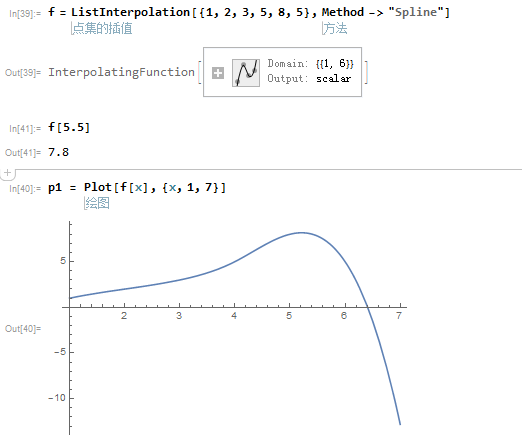## 解决办法

``points = Transpose[{Range, {1, 2, 3, 5, 8, 5}}]``

``````pf[x_] = Piecewise[{
{InterpolatingPolynomial[Take[points, {1, 4}], x], x < 3},
{InterpolatingPolynomial[Take[points, {2, 5}], x], 3 <= x < 4},
{InterpolatingPolynomial[Take[points, {3, 6}], x], x >= 4}
}]``````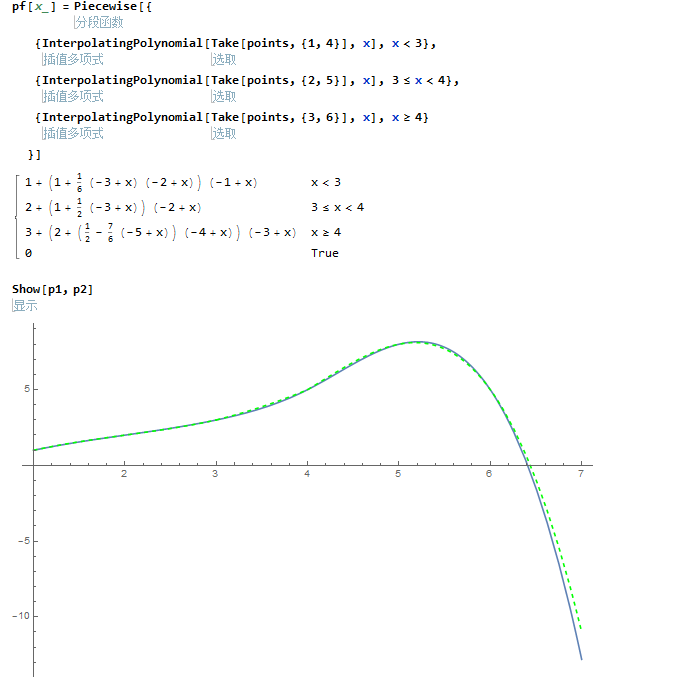### 关于区间的划分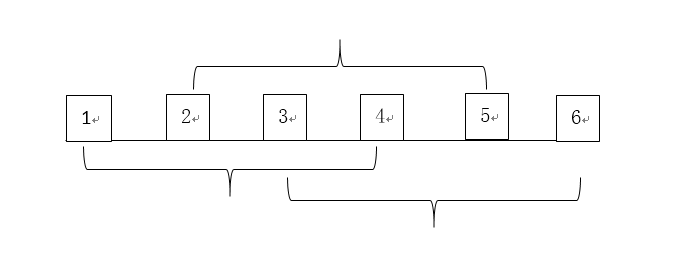## 再看例子

``````time = Table[i, {i, 6, 18, 2}];
tempIn = {18, 20, 22, 25, 30, 28, 24};
points = Transpose[{time, tempIn}];
pf[x_] = Piecewise[{
{InterpolatingPolynomial[Take[points, {1, 4}], x], 6 <= x < 10},
{InterpolatingPolynomial[Take[points, {2, 5}], x], 10 <= x < 12},
{InterpolatingPolynomial[Take[points, {3, 6}], x], 12 <= x < 14},
{InterpolatingPolynomial[Take[points, {4, 7}], x], 14 <= x < 18}
}]``````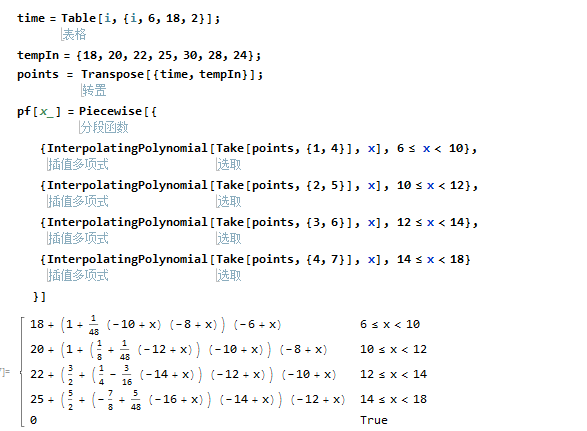``````fIn = Interpolation[Transpose[{time, tempIn}], Method -> "Spline"];
Show[
Plot[fIn[x], {x, 6, 18.5}, ImageSize -> Large],
Plot[pf[x], {x, 6, 18}, PlotStyle -> {Green, Dashed}],
ListPlot[points, PlotStyle -> Hue[.1]]``````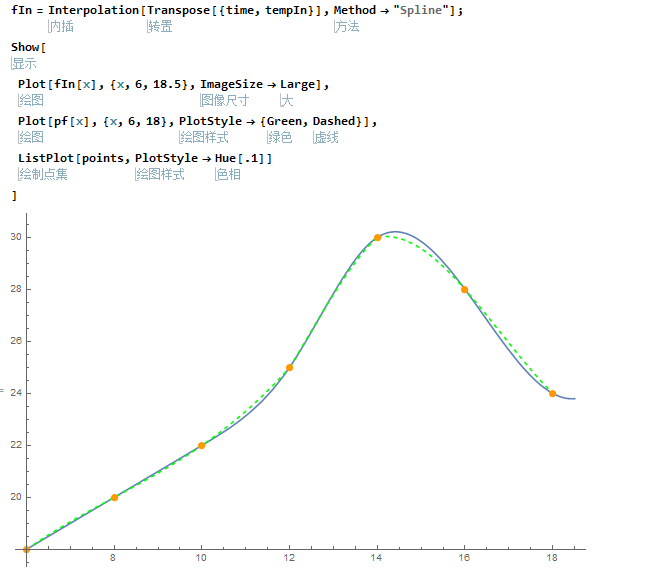• 微信公众号
• 关注微信公众号
•• QQ群
• 我们的QQ群号
•• 本文由 发表于 2017年10月12日15:44:18
• 转载请务必保留本文链接：https://mathpretty.com/8426.html